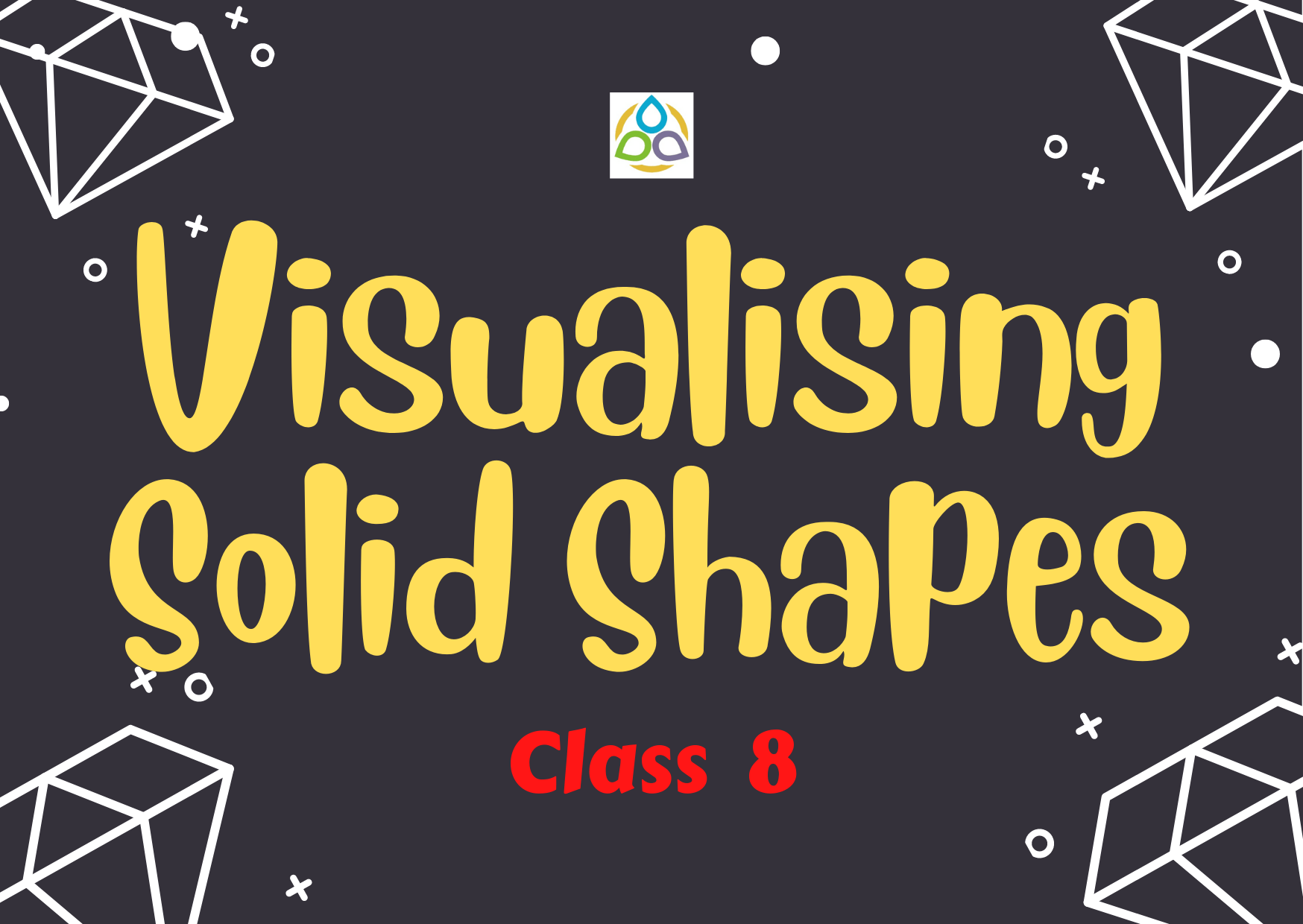# Visualising Solid Shapes – 8

Multiple Choice Questions

73. Solid shapes are of

• (A) Two dimensions
• (B) Three dimensions
• (C) One dimension
• (D) Four dimensions

72. The corners of a solid shape are called its

• (A) Faces
• (B) Edges
• (C) Vertices
• (D) Flat surface

71. The line segments/ curves which form the skeleton of the solid shapes are known as its

• (A) Faces
• (B) Edges
• (C) Vertices
• (D) Flat surface

70. The flat surfaces of a solid shape are called its

• (A) Faces
• (B) Edges
• (C) Vertices
• (D) Flat surface

69. The skeleton outline of a solid that can be folded to make the solid is known as

• (A) Faces
• (B) Edges
• (C) Vertices
• (D) Net

68. A prism is a polyhedron whose lateral faces are

• (A) Circles
• (B) Triangles
• (C) Parallelograms
• (D) Rhombuses or Rhombi

67. A pyramid is a polyhedron whose lateral faces are

• (A) Rectangles
• (B) Triangles
• (C) Parallelograms
• (D) Rhombuses or Rhombi

66. In a regular polyhedron, how many faces meet at each vertex?

• (A) Number of faces > number of vertices
• (B) Number of faces = number of vertices
• (C) Number of faces < number of vertices
• (D) None of these

65. With usual notation, Euler’s formula for any polyhedron is

• (A) F + V + E = 2
• (B) FV + E = 2
• (C) F + VE = 2
• (D) FVE = 2

64. A polyhedron is regular if

• (A) its faces are congruent regular polygons and the same number of faces meet at each vertex
• (B) its faces are congruent regular polygons
• (C) the same number of faces meet at each vertex
• (D) None of these

63. How many edges are there in a pentagonal prism?

• (A) 16
• (B) 15
• (C) 18
• (D) 12

62. How many faces are there in a pentagonal prism?

• (A) 6
• (B) 7
• (C) 8
• (D) 12

61. If a pyramid has a hexagonal base, then the number of vertices is

• (A) 6
• (B) 7
• (C) 8
• (D) 12

60. Which of the following will not form a polyhedron?

• (A) 3 triangles
• (B) 2 triangles and 3 parallelogram
• (C) 8 triangles
• (D) 1 pentagon and 5 triangles

59. Which of the following is a regular polyhedron?

• (A) Cuboid
• (B) Triangular prism
• (C) Cube
• (D) Square prism

58. Which of the following is a two Dimensional figure?

• (A) Rectangle
• (B) Rectangular Prism
• (C) Square Pyramid
• (D) Square Prism